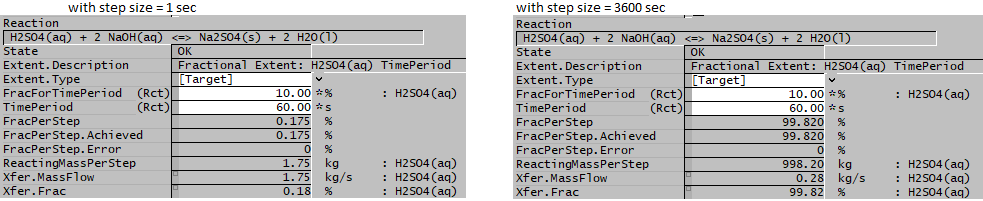# Reaction Block - Extents

Navigation: Models ➔ Sub-Models ➔ Reaction Block (RB) ➔ Extents

Reaction Format RB Data Section RB Sub Model (Model Theory)
Reaction Editor Text File Format Reaction Block
(RB) Summary
Individual Reactions Reaction Extents Source / Sink /
Heat Exchange
Solving Order -
Sequential or Simultaneous
Energy Balance Heat of Reaction / Heat of Dilution / Partial Pressures

# Introduction

There are a number of methods for expressing the extent of a reaction in a Reaction Block. Some of these methods are valid for both Steady State and Dynamic reaction blocks, and some will only be valid in one or the other:

• The term RB, or Reaction Block, is used for steady state projects or in units with no content in Dynamic projects.
• The term CRB, or Content Reaction Block, is used in units with content in Dynamic modelling.
• If the heading has RB and CRB then the method is valid for both types of reaction block, otherwise it is only valid for the type stated.

The image below is from the Reaction Editor: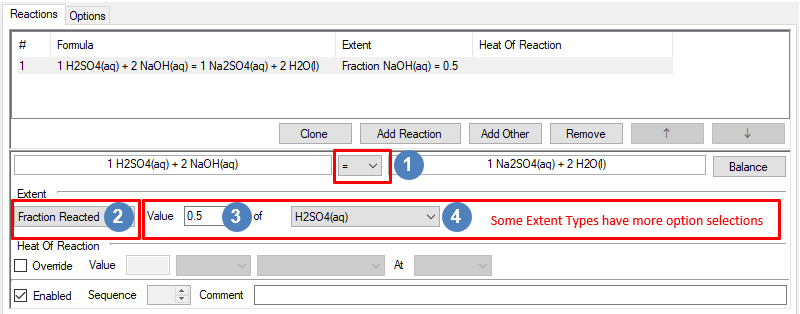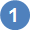The Reaction Direction is discussed here as this is important for the various extent types that can be specified.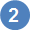Choose the Extent type from the drop down list. These will be explained in detail below.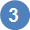For Extent type Fraction Reacted, select the required extent in the Value box. Please note that this value can also be set from the Access Window as this is a "White" Field.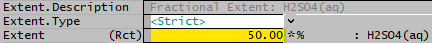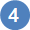For Extent type Fraction Reacted, select the extent species from the dropdown list

NOTE:

## Reaction Directions

-> Forward Reaction

Use this for forward only reactions, particularly used for simultaneous reactions. This also works well when the reaction extent is expressed using the Fraction method.

<-> or = Reversible Reaction

The reaction can proceed either way. This is recommended when using the following extent types:

• Final Concentration
• Final Fraction
• Mass or Molar Ratio
• Equilibrium

If a reversible reaction is not defined for these types of extents then the requirement may not be met as the reaction can only proceed in one direction.

# Specifying Reaction Extent

## Reaction Extent : by Fraction

### Fraction (RB)

The user specifies the fraction of one of the reactants that must react. This fraction of the specified reactant will react provided there are sufficient quantities of all other reactants.

Notes:

1. If the reaction does NOT proceed to the required fraction, this will always be caused by a lack of one of the other reactants or user specified limit (see point 4 below).
2. Reactions using this extent type can be reversible if the user specifies = or <-> in the reaction file. This means that the user may specify a NEGATIVE fractional extent of reaction and this will not cause an error.
3. If the user never wants the reaction to proceed in a negative direction then they must specify a forward only reaction, ->.
4. In SysCAD 9.3 Build 137, user may specify a limit for the reaction extent to avoid fully reacting a species. (This option is switched on from RB Tab - under Settings - LimitFractionReacted, when switched on, extra limit fields will be visible for each individual reactions.)

Example

For the reaction H2SO4(aq) + NaOH(aq) = Na2SO4(s) + H2O(l), the user specifies a fraction of 0.5 for H2SO4(aq).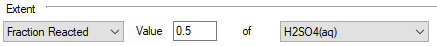If there is sufficient NaOH(aq), 50% of the H2SO4(aq) will react.

### Final Fraction (RB & CRB)

The user specifies a mass or mole fraction of a particular species required at the reaction. The reaction will proceed as required to achieve this fraction provided there are sufficient quantities of all other species.

• The species can be either a reactant or a product.
• The fraction can be specified either as a fraction of all material of the same occurrence (by Phase) or as a fraction of all material (by Total).

Notes:

1. The fraction specified will be achieved at the actual reaction, NOT the fraction in the product from the reaction block.
Therefore, reactions using this extent type should be the last reaction (or be part of the last reaction sequence) in a reaction block. Otherwise the subsequent reactions may change the fraction in the final product. Thus it is advised to only use one of these extent types in each reaction block.
2. The specification of by Phase or by Total is optional. If this is not specified then by Phase is assumed.
3. Reactions using this extent type are normally reversible, i.e. use = or <-> when specifying the reaction direction. This will allow SysCAD to achieve the required final fraction, provided there are sufficient quantities of all other species. If the user specifies a forward only (->) reaction, then the final fraction may not be achieved.
4. It is not advised to have more than one reaction with this extent type in a set of simultaneous reactions. This may lead to situations which have no solution or multiple solutions and may also reduce the speed of convergence.
5. In SysCAD 9.3 Build 137, user may specify a limit for the reaction extent to avoid fully reacting a species. (This option is switched on from RB Tab - under Settings - LimitFractionReacted, when switched on, extra limit fields will be visible for each individual reactions.) This limit may affect the actual extent achieved.

Example

For the reaction H2SO4(aq) + NaOH(aq) = Na2SO4(s) + H2O(l), the user specifies a Final Mass Fraction of Na2SO4(s) of 0.1 by Phase.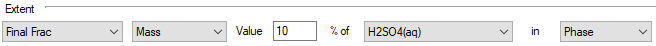If there are sufficient quantities of the required species then the reaction will proceed (in either direction as the reaction is reversible) in order to achieve a final mass fraction of 10% of Na2SO4(s) in the Solid phase.

## Reaction Extent : by Concentration

### Final Concentration (RB & CRB)

NOTE: mole concentration is only available in SysCAD 9.3 Build 137 or later.

The user specifies the concentration of a particular species required at the reaction. The reaction will proceed as required to achieve this concentration provided there are sufficient quantities of all other species.

• The species can be either a reactant or a product.
• The concentration is specified in grams (or mole) of the species per litre of material of liquid phase.
• The user can optionally specify a temperature (in °C) at which the concentration is to be calculated. If no temperature is specified, then the default is the product temperature.

Notes:

1. The concentration specified will be achieved at the actual reaction, NOT the concentration in the product from the reaction block.
• Therefore, reactions using this extent type should be the last reaction (or be part of the last reaction sequence) in a reaction block. Otherwise the subsequent reactions may change the concentration achieved. Thus it is advised to only use one of these extent types in each reaction block.
2. Reactions using this extent type are normally reversible, i.e. use = or <-> when specifying the reaction direction. This will allow SysCAD to achieve the required final concentration, provided there are sufficient quantities of all other species.
3. If the user specifies a forward only (->) reaction, then the final concentration may not be achieved.
4. It is not advised to have more than one reaction with this extent type in a set of simultaneous reactions. This may lead to situations which have no solution or multiple solutions and may also reduce the speed of convergence.

Example

For the reaction H2SO4(aq) + NaOH(aq) = Na2SO4(s) + H2O(l), the user specifies a final concentration of 5.5g/L of H2SO4(aq) at 25°C.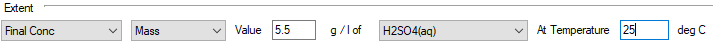If there are sufficient quantities of the required species then the reaction will proceed (in either direction as the reaction is reversible) in order to achieve a final concentration of 5.5 grams of H2SO4(aq)/Litre of Liquid at 25 °C.

### Final Concentration based on Individual Phase (RB & CRB)

NOTE: This method is only available in SysCAD 9.3 Build 137 or later.

The user specifies the mass or mole of a particular species per required individual phase for the reaction. The reaction will proceed as required to achieve this concentration provided there are sufficient quantities of all other species.

• The species can be either a reactant or a product.
• The concentration is specified in grams or mole of the species per litre of material of specified individual phase.
• The user can optionally specify a temperature (in °C) at which the concentration is to be calculated. If no temperature is specified, then the default is the product temperature.

Notes:

1. The concentration specified will be achieved at the actual reaction, NOT the concentration in the product from the reaction block.
• Therefore, reactions using this extent type should be the last reaction (or be part of the last reaction sequence) in a reaction block. Otherwise the subsequent reactions may change the concentration achieved. Thus it is advised to only use one of these extent types in each reaction block.
2. The aqueous phase (aq) includes water (defined as H2O(l)). The liquid phase (l) excludes water (defined as H2O(l)). So please take care when selecting the individual phase from the list. See example below.
3. Reactions using this extent type are normally reversible, i.e. use = or <-> when specifying the reaction direction. This will allow SysCAD to achieve the required final concentration, provided there are sufficient quantities of all other species.
4. If the user specifies a forward only (->) reaction, then the final concentration may not be achieved.
5. It is not advised to have more than one reaction with this extent type in a set of simultaneous reactions. This may lead to situations which have no solution or multiple solutions and may also reduce the speed of convergence.

Example

1. For the reaction H2SO4(aq) + NaOH(aq) = Na2SO4(s) + H2O(l), the user specifies a final concentration of 5.5g/L of H2SO4(aq) in aq at 25°C.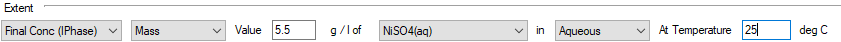• If there are sufficient quantities of the required species then the reaction will proceed (in either direction as the reaction is reversible) in order to achieve a final concentration of 5.5 grams of H2SO4(aq)/Litre of aqueous phase (including water) at 25 °C.
2. For the reaction FeO(s) = FeO(sl), the user specifies a final concentration of 1 g/L FeO(sl) in slag at 25°C.
• If there are sufficient quantities of the required species then the reaction will proceed (in either direction as the reaction is reversible) in order to achieve a final concentration of 1 grams of FeO in the slag phase /Litre of slag species at 25 °C.

### Final Elemental Concentration (RB & CRB)

NOTE: This method is only available in SysCAD 9.3 Build 137 or later.

The user specifies the mass or mole of a particular element in a required individual phase per Liquid phase for the reaction. The reaction will proceed as required to achieve this concentration provided there are sufficient quantities of all other species.

• The concentration is specified in grams (or mole) of the element in a required individual phase per litre of material of liquid phase.
• The user can optionally specify a temperature (in °C) at which the concentration is to be calculated. If no temperature is specified, then the default is the product temperature.

Notes:

1. The concentration specified will be achieved at the actual reaction, NOT the concentration in the product from the reaction block.
• Therefore, reactions using this extent type should be the last reaction (or be part of the last reaction sequence) in a reaction block. Otherwise the subsequent reactions may change the concentration achieved. Thus it is advised to only use one of these extent types in each reaction block.
2. Reactions using this extent type are normally reversible, i.e. use = or <-> when specifying the reaction direction. This will allow SysCAD to achieve the required final concentration, provided there are sufficient quantities of all other species.
3. If the user specifies a forward only (->) reaction, then the final concentration may not be achieved.
4. It is not advised to have more than one reaction with this extent type in a set of simultaneous reactions. This may lead to situations which have no solution or multiple solutions and may also reduce the speed of convergence.

Example

For the reaction H2SO4(aq) + NaOH(aq) = Na2SO4(s) + H2O(l), the user specifies a final concentration of 1 g/L of S(aq) at 25°C.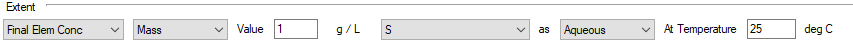If there are sufficient quantities of the required species then the reaction will proceed (in either direction as the reaction is reversible) in order to achieve a final elemental concentration of 1 grams of S in aqueous phase /Litre of Liquid at 25 °C.

### Final Elemental Concentration based on Individual Phase (RB & CRB)

NOTE: This method is only available in SysCAD 9.3 Build 137 or later.

The user specifies the mass or mole of a Element in a required phase per required individual phase for the reaction. The reaction will proceed as required to achieve this concentration provided there are sufficient quantities of all other species.

• The concentration is specified in gram or mole of the element of the chosen phase per litre of material of specified individual phase.
• The user can optionally specify a temperature (in °C) at which the concentration is to be calculated. If no temperature is specified, then the default is the product temperature.

Notes:

1. The concentration specified will be achieved at the actual reaction, NOT the concentration in the product from the reaction block.
• Therefore, reactions using this extent type should be the last reaction (or be part of the last reaction sequence) in a reaction block. Otherwise the subsequent reactions may change the concentration achieved. Thus it is advised to only use one of these extent types in each reaction block.
2. The aqueous phase (aq) includes water (defined as H2O(l)). The liquid phase (l) excludes water (defined as H2O(l)). So please take care when selecting the individual phase from the list. See example below.
3. Reactions using this extent type are normally reversible, i.e. use = or <-> when specifying the reaction direction. This will allow SysCAD to achieve the required final concentration, provided there are sufficient quantities of all other species.
4. If the user specifies a forward only (->) reaction, then the final concentration may not be achieved.
5. It is not advised to have more than one reaction with this extent type in a set of simultaneous reactions. This may lead to situations which have no solution or multiple solutions and may also reduce the speed of convergence.

Example

1. For the reaction H2SO4(aq) + NaOH(aq) = Na2SO4(s) + H2O(l), the user specifies a final concentration of 1 g/L of S(aq) in aq phase at 25°C.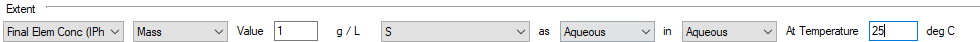• If there are sufficient quantities of the required species then the reaction will proceed (in either direction as the reaction is reversible) in order to achieve a final concentration of 1 grams of elemental sulphur in aqueous phase /Litre of aqueous species (including water) at 25 °C.
2. For the reaction FeO(s) = FeO(sl), the user specifies a final concentration of 1 g/L Fe(sl) in slag at 25°C.
• If there are sufficient quantities of the required species then the reaction will proceed (in either direction as the reaction is reversible) in order to achieve a final concentration of 1 grams of elemental Fe in the slag phase /Litre of slag species at 25 °C.

## Reaction Extent : by Flow

### Amount Reacted (RB & CRB)

NOTE: This method is only available in SysCAD 9.3 Build 137 or later.

The user specifies the mass or mole flow of one of the reactants that must react. The required flow of the specified reactant will react provided there are sufficient quantities of all reactants.

Notes:

1. If the reaction does NOT proceed to the required fraction, this will be caused by a lack of one of the reactants (including the specified reactant).
2. Reactions using this extent type cannot be reversible.
3. User may specify a limit for the reacting mass/moles. (This option is switched on from RB Tab - under Settings - LimitAmountReacted, when switched on, extra limit fields will be visible for each individual reactions.)

Example

For the reaction H2SO4(aq) + NaOH(aq) = Na2SO4(s) + H2O(l), the user specifies a mass flow of 5 kg/h for H2SO4(aq).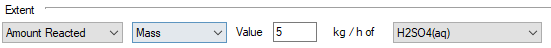If there is sufficient NaOH(aq), 5 kg/h of the H2SO4(aq) will react.

### Final Flow (RB only)

NOTE: This method is only available in SysCAD 9.3 Build 137 or later.

The user specifies the mass or mole flow of a particular species required at the end of the reaction step. The reaction will proceed as required to achieve this flow provided there are sufficient quantities of all other species.

• The species can be either a reactant or a product.

Notes:

1. The flow specified will be achieved at the end of the current reaction step, NOT the product from the reaction block.
if the specified species is used in multiple reactions, subsequent use of the species after the current reaction step may affect the flow calculated by the reaction.
2. Reactions using this extent type are normally reversible, i.e. use = or <-> when specifying the reaction direction. This will allow SysCAD to achieve the required final flow, provided there are sufficient quantities of all other species.
3. If the user specifies a forward only (->) reaction, then the final flow may not be achieved.
4. It is possible to have more than one reaction with this extent type in a reaction block, provided the required species is different and only used once per reaction.
5. User may specify a limit for the reacting mass/moles. (This option is switched on from RB Tab - under Settings - LimitAmountReacted, when switched on, extra limit fields will be visible for each individual reactions.)

Example

For the reaction H2SO4(aq) + NaOH(aq) = Na2SO4(s) + H2O(l), the user specifies a final flow of 0.001 kg/h of H2SO4(aq).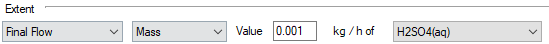If there are sufficient quantities of the required species then the reaction will proceed (in either direction as the reaction is reversible) in order to achieve a final flow of 1 g/h of H2SO4(aq).

## Reaction Extent : Other

### Ratio (RB & CRB)

The user specifies the required molar or mass ratio of a Product to a Reactant. The Ratio = Product/Reactant.

The reaction will proceed as required to achieve this ratio provided there are sufficient quantities of all required species.

Notes:

1. Reactions using this extent type are normally reversible, i.e. use = or <-> when specifying the reaction direction. This will allow SysCAD to achieve the required ratio, provided there are sufficient quantities of all other species. If the user specifies a forward only (->) reaction, then the ratio may not be achieved.
2. Reactions using this extent type should be the last reaction (or be part of the last reaction sequence) in a reaction block. Otherwise the subsequent reactions may change the ratio achieved. Thus it is advised to only use one of these extent types in each reaction block.

Example

For the reaction H2SO4(aq) + NaOH(aq) = Na2SO4(aq) + H2O(l), the user specifies a Mass Ratio of H2SO4(aq) : Na2SO4(aq) = 1:20.
If there are sufficient quantities of the required species then the reaction will proceed (in either direction as the reaction is reversible) in order to achieve a final mass ratio of H2SO4(aq) to Na2SO4(aq) of 1:20, i.e. Na2SO4(aq) = 20 * H2SO4(aq).

### Equilibrium (RB & CRB)

The user specifies the Equilibrium constant (K) for a reaction. For liquid reactions this is Kf, for gaseous reactions this is Kp. The reaction will proceed as required to achieve equilibrium provided there are sufficient quantities of all other species.

Consider a reaction of the form: aA + bB = cC + dD

For liquid reactions:

$\displaystyle{ K_f = \cfrac{[C]^c [D]^d}{[A]^a [B]^b} }$
Where [X] = molar concentration of species X in mol/L.
Solids are not included in the equilibrium equation
Water is not included in the equilibrium equation.

For gaseous reactions:

$\displaystyle{ K_p = \cfrac{(Cpp)^c (Dpp)^d}{(App)^a (Bpp)^b} }$
Where Xpp = partial pressure of species X, in kPa.
Solids are not included in the equilibrium equation.

Notes:

1. Reactions which contain only solid species can not use this extent type.
2. Reactions which contain both liquid and gaseous species can not use this extent type.
3. Reactions using this extent type are normally reversible, i.e. use = or <-> when specifying the reaction direction. This will allow SysCAD to achieve the required equilibrium, provided there are sufficient quantities of all other species. If the user specifies a forward only (->) reaction, then equilibrium may not be achieved.
4. Reactions using this extent type should be the last reaction (or be part of the last reaction sequence) in a reaction block. Otherwise the subsequent reactions may change the equilibrium achieved. Thus it is advised to only use one of these extent types in each reaction block.

Example:

For the dissociation of CaSO4(s) = Ca2+ + SO42-, the equilibrium constant ~ 2e-5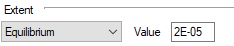## Reaction Extent : Calculations and Properties

### User Defined Properties (User Calc) (RB and CRB)

NOTE: This method is only available in SysCAD 9.3 Build 137 or later.

This requires User calculations - User Properties to be present in the project. The user calculations are defined as part of the project configuration file, please see User Property Calculations for more information.

If a user property is present, the user may select the required property to control the reaction extent.

If a user property is not present in the project, then <No UserCalcs Available> will be displayed in the selection box of the reaction editor.

Notes:

1. Not all user defined properties can be used for reaction extent, please make sure the user property selected is related to the selected reaction.

Example

For the Gold Example project, we can replace the last reaction with User Calc of CuonCarbon_ppm. This way, the reaction extent will be adjusted based on the user calculated ratio or Cu:C.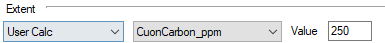### User Defined Species Calculation (Sp Calc) (RB and CRB)

NOTE: This method is only available in SysCAD 9.3 Build 137 or later.

This requires User calculations - User Species Calculation to be present in the project. The user calculations are defined as part of the project configuration file, please see Species Calculations for more information.

If a user species calculation is present, the user may select the required calculation to control the reaction extent.

If a user species calculation is not present for the project, then <No SpCalcs Available> will be displayed in the selection box of the reaction editor.

Notes:

1. Not all user defined species calculation can be used for reaction extent, please make sure the user calculation selected is related to the selected reaction.

Example

For the Nickel Copper Project, we can specify reaction extent in terms of Fe3+ or Fe2+.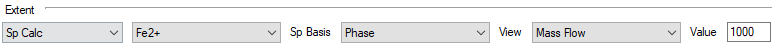### Species Model Properties (Sp Model Prop) (RB and CRB)

NOTE: This method is only available in SysCAD 9.3 Build 137 or later.

This allows the user to control the reaction extent based on selected species model properties.

Examples

For the standard species model, user can use properties such as TSS (total suspended solids), TDS (total dissolved solids) to control the solution impurities.
For the Alumina species model, user can specify the Gibbsite Dissolution reaction in terms of the A/C: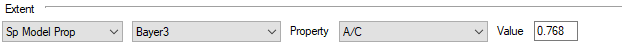## Reaction Extent: Dynamic

### Dynamic Rate (CRB)

This method can only be used for Dynamic simulation unit operations with content.

The user specifies both a fraction and a fractional rate at which one of the reactants will react. The actual fraction of the reactant to be reacted in a given iteration is the product of the fraction, the fractional rate and the stepsize (in seconds). This fraction of the specified reactant will react provided there are sufficient quantities of all other reactants.

The user may choose to have the rate 'Stabilised'. This means the rate is a first order response and the fraction reacted is given by: Fraction * (1- exp(-1 * Rate * DeltaTime))

Notes:

1. Reactions using this extent type can be reversible if the user specifies = or <-> in the reaction file. This means that the user may specify a NEGATIVE fractional extent of reaction and this will not cause an error. If the user does not want to have the reaction proceed in a negative direction then they must specify a forward only reaction, ->.
2. The dynamic rate option is only able to be used when the user has chosen for the reactions to occur on the content of the unit by turning on a reaction in the Content Evaluation Block (CEB).

Example

For the reaction H2SO4(aq) + NaOH(aq) = Na2SO4(s) + H2O(l), the user specifies a fraction of 0.1 (ie 10%) and a fractional rate of 0.05 (ie 5 %/s) for H2SO4(aq).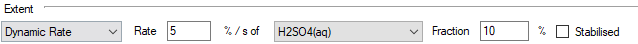If there is sufficient NaOH(aq) then the rate of reaction of H2SO4(aq) will be 0.1 * 0.05 * iteration size (in seconds).
A) If the project is running with an iteration size of 1 second, then the rate of reaction of H2SO4(aq) = 0.1 * 0.05 * 1 = 0.005, or 0.5% per second.
B) If the project is running with an iteration size of 1 minute, then the rate of reaction of H2SO4(aq) = 0.1 * 0.05 * 60 = 0.3, or 30% per minute.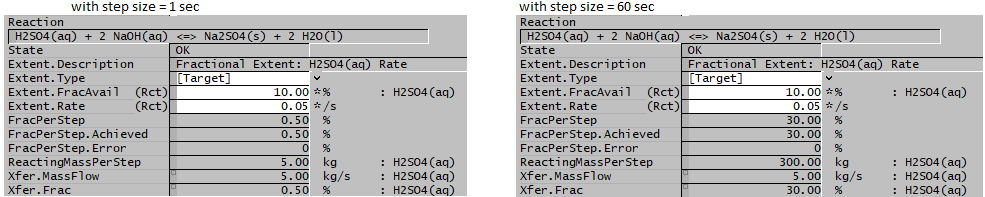### Dynamic Fraction (CRB)

This method can only be used for Dynamic simulation unit operations with content.

The user specifies a fraction and a time period in which this fraction of the reactant will react. The fraction of the reactant to be reacted in a given iteration is calculated by the following formula:

$\displaystyle{ (1-r)^n = 1-R }$
where
Smaller Time Period = minimum(Time Period, Step Size)
Larger Time Period = maximum(Time Period, Step Size)
n = Larger Time Period / Smaller Time Period
r = Fractional extent at the smaller time period
R = Fractional extent at the larger time period

This fraction of the specified reactant will react provided there are sufficient quantities of all other reactants.

Notes:

1. Reactions using this extent type can be reversible if the user specifies = or <-> in the reaction file. This means that the user may specify a NEGATIVE fractional extent of reaction and this will not cause an error. If the user does not want to have the reaction proceed in a negative direction then they must specify a forward only reaction, ->.
2. The dynamic rate option is only able to be used when the user has chosen for the reactions to occur on the content of the unit by turning on a reaction in the Content Evaluation Block (CEB).

Example

For the reaction H2SO4(aq) + NaOH(aq) = Na2SO4(s) + H2O(l), the user specifies a fraction of 0.1 in a time period of 60 seconds for H2SO4(aq).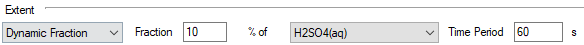If there is sufficient NaOH(aq) then
A) if the project is running with an iteration size of 1 second, then n = 60/1 = 60, R = 0.1 and hence the rate of reaction of H2SO4(aq) (r) = 0.1754% per iteration.
B) if the project is running with an iteration size of 1 hour, then n = 3600/60 = 60, r = 0.1 and hence the rate of reaction of H2SO4(aq) (R) = 99.82% per iteration.